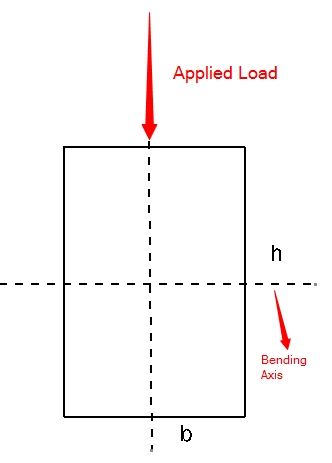This is the Solid Beam (Rectangular) Moment of Inertia Calculator. Simply enter the length of the two side of the rectangular beam to find the moment of inertia.b = h = b is the length of the side of the rectangle which is always parallel to bending axis.h is the length of the side of the rectangle which is always perpendicular to bending axis.